Courses

# Friction Force MCQ Level - 1

## 10 Questions MCQ Test Topic wise Tests for IIT JAM Physics | Friction Force MCQ Level - 1

Description
This mock test of Friction Force MCQ Level - 1 for Physics helps you for every Physics entrance exam. This contains 10 Multiple Choice Questions for Physics Friction Force MCQ Level - 1 (mcq) to study with solutions a complete question bank. The solved questions answers in this Friction Force MCQ Level - 1 quiz give you a good mix of easy questions and tough questions. Physics students definitely take this Friction Force MCQ Level - 1 exercise for a better result in the exam. You can find other Friction Force MCQ Level - 1 extra questions, long questions & short questions for Physics on EduRev as well by searching above.
QUESTION: 1

### What is the minimum stopping distance for a vehicle of mass m moving with speed v along a level road. If the coefficient of friction between the tyres and the road is μ.

Solution: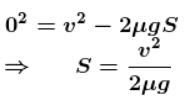The correct answer is: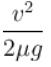QUESTION: 2

### Car is accelerating with acceleration is 20m/s2. A box of mass m = 10kg that is placed inside the car, it is put in contact with the vertical wall of car as shown. The friction coefficient between the box and the wall is μ = 0.6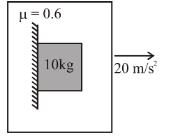Solution:

The breaking force is insufficient, so the block will not slide.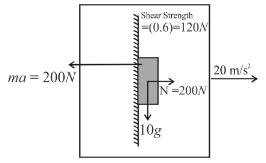So friction force = 100 N
and acceleration will be 20 m/sec2
Net contact force on the block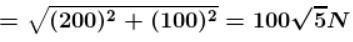The correct answer is: The friction force acting on the box will be 100 N

QUESTION: 3

### A horizontal force of 10N  is necessary to just hold a block stationary against a wall. The coefficient of friction between the block and the walls is 0.2. The weight of the block is  (g =10m/s2)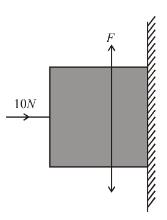Solution:

Force F = μR = mg
Weight of block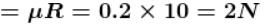The correct answer is: 2 N

QUESTION: 4

A bead of mass m  is located on a parabolic wire with its axis vertical and vertex directed towards downwards as in figure and whose equation is x2 = ay. If the coefficient of friction is μ  the highest distance above the x-axis at which the particle will be in equilibrium is :

Solution:

For the sliding not to occur when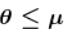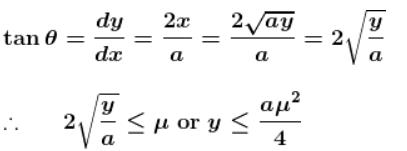QUESTION: 5

A block A of mass 2 kg rest on another block B of mass 8 kg which rests on a horizontal floor. The coefficient of friction between A and B is 0.2 while that between B and floor is 0.5. When a horizontal force of 25 N is applied on the block B. The force of friction between A and B is :

Solution: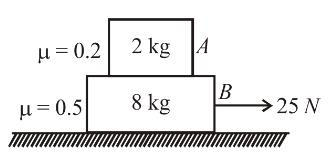frictional force on B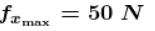B
will not slide on ground. So force = 0.

QUESTION: 6

A smooth block is released at rest on a 45° incline and then slides a distance d.  The time taken to slide is n times as much to slide on rough incline then on a smooth incline. The coefficient of friction is :

Solution:

When friction is absent a1 = g sin θ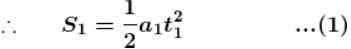When friction is present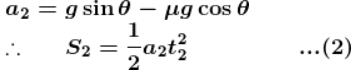From Eq. (1) and (2)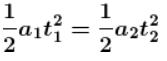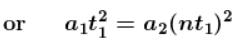(∴ t2 = nt1)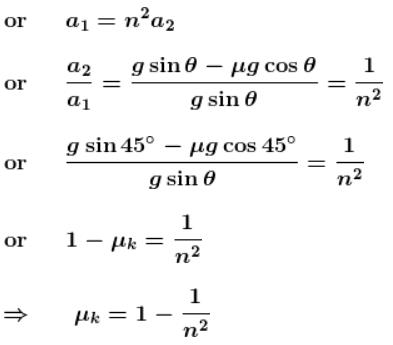The correct answer is: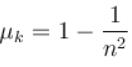QUESTION: 7

A weight w is to be moved from the bottom to the top of an inclined plane of inclination θ to the horizontal. If a smaller force is to be applied to drag it along the plane is comparison to lift it vertically up, the coefficient of friction should be such that :

Solution: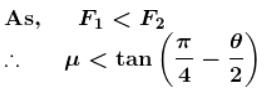The correct answer is: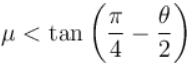QUESTION: 8

A chain of length L is placed on a horizontal surface as shown in figure. At any instant x  is the length of chain on rough surface and the remaining portion lies on smooth surface. Initially  x = 0, a horizontal force P is applied to the chain (as shown in figure). In the duration  x  changes from  x = 0 to  x = L, for chain to move with constant speed.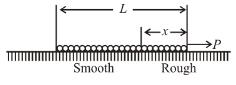Solution:

For chain to move with constant speed P needs to be equal to frictional force on the chain. As the length chain on the rough surface increases. Hence, the friction force fk = μkN increase.
Hence, magnitude of P should increase with time.
The correct answer is: The magnitude of P should increase with time

QUESTION: 9

A box ‘A’ is lying on the horizontal floor of the compartment of a train running along horizontal rails from left to right. At time ‘t’, it decelerates. Then the resultant contact force R by the floor on the box is given best by :

Solution: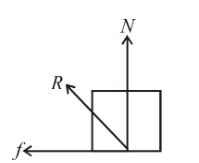Acceleration of train will be from right to left.
⇒ Pseudo force will act on the box from left to right therefore friction will act from right to left.
The correct answer is: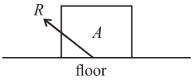QUESTION: 10

If the coefficient of friction between A and B is  μ the maximum horizontal acceleration of the wedge A for which B will remain at rest with respect to the wedge is :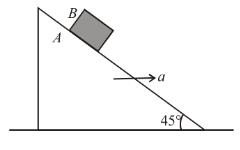Solution:

FBD of block B w.r.t. wedge A, for maximum a perpendicular to wedge :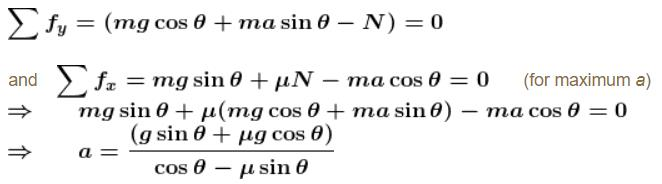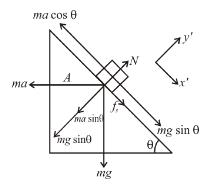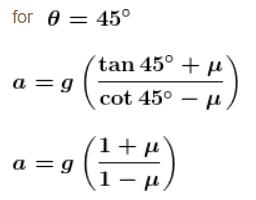The correct answer is: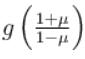Track your progress, build streaks, highlight & save important lessons and more!

### Similar Content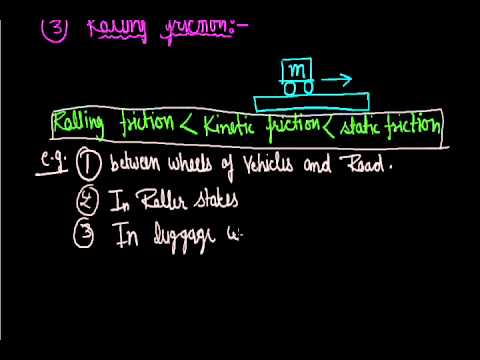### Related tests